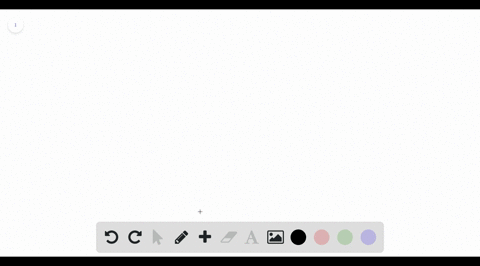Black Friday is Here! Start Your Numerade Subscription for 50% Off!Join Today### Refer to the figure and find the volume generated…

00:59Problem 23

# Refer to the figure and find the volume generated by rotating the given region about the specified line.$\Re_2$ about $OA$

## Discussion

You must be signed in to discuss.

## Video Transcript

we know that we're using the disk and washing method to integrate along the access parallel to the axis of rotation. So the outer radius is gonna be one minus year, which is one cause the distance from Y equals one of the axis of rotation, which is why I call zero. And the inner radius is gonna be the 432 acts again for the same reasons. Distance to the axis of rotation. Therefore, our volume is pi times the integral from 01 outer, which is ones, and it's squared minus inner 432 ax squared D X integrate, which means use the power rule. Increase the experiment by wand and divide by the new exponents. And now we can plug in our values zero and one or two bounds. As you can see, we end up with pi over three